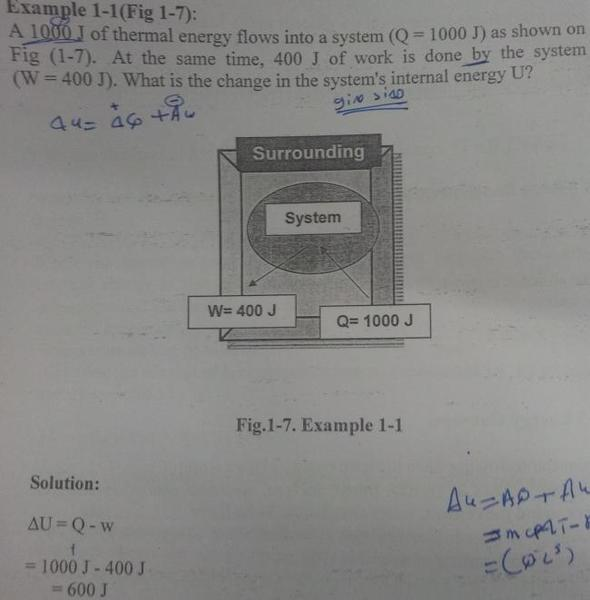# Thermodynamics when work being negative or positive

manal950
Hi

How are you

I have a question how we can know the work if - or +

also heat energy

please could see this question why in answer
take work as -
and heat as +## Answers and Replies

Homework Helper
hi manal950!I have a question how we can know the work if - or +

it's really english rather than physics …

work done by a system is minus

work done on a system (or to a system) is plusthe question says "400 J of work is done by the system", so the work comes out of the system, so it's minus

(if the question said "400 J of work is done on the system", then the work would come into the system, so it would be plus)​

Staff Emeritus
Homework Helper
Tim got it backwards for the sign convention you're using. You have the first law written as ##\Delta U = Q-W##. Assume for a moment that Q=0. If work is done by the system, the system is expending energy on its surroundings, so the system's energy U must decrease. For this to happen, you need W>0. Similarly, if the surroundings do work on the system, energy is being added to the system, so you want W<0 so that ##\Delta U>0##.

manal950
thanks so much

thanks tiny-tim
thanks vela

Basic_Physics
If the work was negative, that is -400J, then the answer would have been

ΔU = Q - W
= 1000 J - (-400 J)
= 1000 + 400
= 1400 J

So negative work means that the system gained energy or an external force did work on the system.
In this case the problem states that the work is positive 400 J. Which means that the system did work
and therefore lost energy indicated by a lower change in its internal energy.